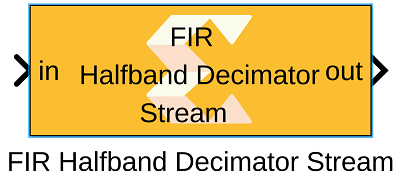# FIR Halfband Decimator Stream - 2022.1 English

## Vitis Model Composer User Guide (UG1483)

Document ID
UG1483
Release Date
2022-05-26
Version
2022.1 English## Library

AI Engine/DSP/Stream IO

## Description

This block implements the stream-based FIR Halfband Decimator filter targeted for AI Engines.

## Parameters

Main
Input data type/Output data type
Describes the type of individual data samples input to and output from the filter function. int16, cint16, int32, cint32, float, cfloat.
Filter coefficients data type
Describes the type of individual coefficients of the filter taps. It should be one of int16, cint16, int32, cint32, float, cfloat and must also satisfy the following rules:
• Complex types are only supported when the Input/Output data type is also complex.
• 32-bit types are only supported when the Input/Output data type is also a 32-bit type.
• Filter coefficients data type must be an integer type if the Input/Output data type is an integer type.
• Filter coefficients data type must be a float type if the Input/Output data type is a float type.
Specify filter coefficients via input port
When this option is enabled, the tool allows you to specify reloadable filter coefficients via input port.
Filter coefficients
Specifies the filter coefficients as a vector of (N+1)/4+1 elements, where 'N' is a positive integer that represents the filter length and must be in the range 4 to 240 inclusive.
Input frame size (Number of samples)
Describes the number of samples used as an input to the filter function. The number of values in the output window will be the Input window size divided by two by virtue of the halfband decimation factor.
Scale output down by 2^
Describes the power of 2 shift down applied to the accumulation of FIR terms before output. It must be in the range 0 to 61.
Rounding mode
Describes the selection of rounding to be applied during the shift down stage of processing. The rounding options are as follows:
1. Floor (truncate)
2. Ceiling
3. Round to positive infinity
4. Round to negative infinity
5. Round symmetrical to infinity
6. Round symmetrical to zero
7. Round convergent to even
8. Round convergent to odd

Modes 2 to 7 round to the nearest integer. They differ only in how they round for the value of 0.5.